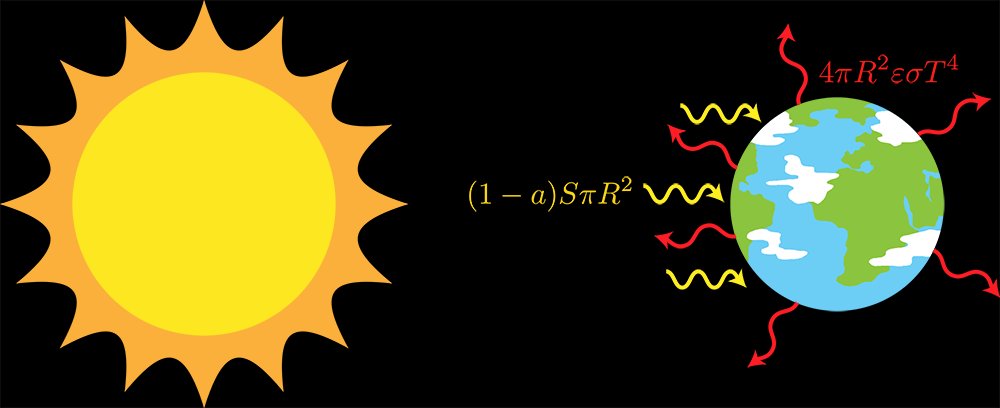Welcome to Project Lovelace! We're still in early development so there are still tons of bugs to find and improvements to make. If you have any suggestions, complaints, or comments please let us know on Discourse, Discord, or GitHub!

# Rock star climate

## You will learn about: the simplest climate model.

In this first problem on climate modeling we'll look at the simplest possible climate model of the Earth and use it to calculate Earth's temperature.

It's a zero-dimensional energy balance model where we'll assume that the energy absorbed by the Earth is equal to the energy being radiated out by the Earth. We can call this balanced state radiative equilibrium where $$\text{Energy in} = \text{Energy out}$$ We can call this model the rock-star model because we have a star that's heating up a rocky planet. The planet has no atmosphere, it's just a rock floating through space. We can use it to calculate the Earth's average temperature.The solar energy being absorbed by the Earth (yellow arrows) is equal to the thermal energy radiated out to space by the Earth (red arrows). The sun only shines on one half of the Earth at a time, while the Earth is radiating energy all over its surface. Not to scale of course!

## Calculating energy in

The solar power hitting the top of the atmosphere is given by the solar constant $S = 1361 \; \mathrm{W/m}^2$. It's very high at the top of the atmosphere but a lot of it gets absorbed by the atmosphere, especially by clouds, so the solar power hitting the Earth's surface is lower than $S$.

The sun shines on only half of the Earth at a time so all that solar power is incident on a circular area $\pi R^2$ where $R$ = 6371 km is the radius of the Earth. So the total solar energy reaching the Earth is $S\pi R^2$.

But some of the solar energy is reflected back to space by stuff like clouds, snow, and sand. The fraction reflected back is called the albedo $a$ which is between 0 (everything is absorbed) and 1 (everything is reflected). Clouds and snow have a pretty high albedo while forests and the ocean have a pretty low albedo, but on average Earth's albedo is $a \approx 0.306$.

So the total energy absorbed by the Earth is $$\text{Energy in} = (1 - a) S \pi R^2$$

## Calculating energy out

We can treat the Earth as a gray body that radiates thermal energy (or heat) everywhere on its surface according to the Stefan-Boltzmann law $\varepsilon \sigma T^4$ where $\sigma = 5.670374419 \times 10^{-8} \mathrm{W} \cdotp \mathrm{m}^{-2} \cdotp \mathrm{K}^{-4}$ is the Stefan-Boltzmann constant and $T$ is the temperature of the Earth.

The emissivity $\varepsilon$ is a measure of how good a body is at emitting thermal energy and is also a number between 0 (perfect absorber that emits no heat) and 1 (perfect emitter). The emissivity of the Earth varies but on average it's $\varepsilon \approx 0.612$.

This energy is being emitted everywhere on the Earth's surface so to get the total energy emitted we multiply by the surface area of the Earth $4\pi R^2$ to get $$\text{Energy out} = 4\pi R^2 \varepsilon \sigma T^4$$

## Calculating the Earth's temperature

From $\text{Energy in} = \text{Energy out}$ we get the following balance $$(1 - a) S \pi R^2 = 4\pi R^2 \varepsilon \sigma T^4$$ so we can solve for T to get $$T = \sqrt{\frac{(1 - a) S}{4\varepsilon\sigma}}$$ which gives the Earth's temperature in degrees Kelvin.

Input: A solar constant $S$ in $\mathrm{W}/\mathrm{m}^2$, albedo $0 \le a \le 1$, and an emissivity $0 \le \varepsilon \le 1$.

Output: The temperature of the rocky planet in Celsius (°C). Remember to convert from Kelvin $K$ to Celsius $C$ using $C = K - 273.15$

Example (Earth)

Input solar constant: 1361 Input albedo: 0.306 Input emissivity: 0.612 Output rock temperature: 14.059374
 Difficulty rock_temperature(solar_constant, albedo, emissivity)

You must be logged in to view your submissions.

### Notes

• The average temperature of the Earth is actually ~14°C so this simple model does pretty well! Of course, this is probably because you can tune the albedo $a$ and emissivity $\varepsilon$ to get a better answer and it's pretty hard to assign the Earth an "average albedo" as it's made up of so many different surfaces, snow and sea ice keep forming and thawing, etc.

### References

A new, lower value of total solar irradiance: Evidence and climate significance, Greg Kopp & Judith L. Lean, Geophysical Research Letters 38(1), 2011.
They measured the most accurate value of the solar constant during the 2008 solar minimum period to be 1360.8 ± 0.5 W/m² which is where we got the value of 1361 W/m².

Let us know what you think about this problem! Was it too hard? Difficult to understand? Also feel free to discuss the problem, ask questions, and post cool stuff on Discourse. You should be able see a discussion thread below. Would be nice if you don't post solutions in there but if you do then please organize and document your code well so others can learn from it.